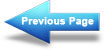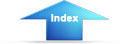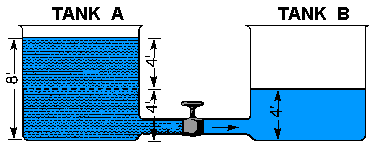Custom SearchMagnetic ShieldingMatter, Energy, and ElectricityVoltage Produced by FrictionElectrical Charges

From the previous study of electrostatics, you learned that a field of force exists in the space surrounding any electrical charge. The strength of the field is directly dependent on the force of the charge.

The charge of one electron might be used as a unit of electrical charge, since charges are created by displacement of electrons; but the charge of one electron is so small that it is impractical to use. The practical unit adopted for measuring charges is the COULOMB, named after the scientist Charles Coulomb. One coulomb is equal to the charge of 6,280,000,000,000,000,000 (six quintillion two hundred and eighty quadrillion) or (6.28 x 1018) electrons.

When a charge of one coulomb exists between two bodies, one unit of electrical potential energy exists, which is called the difference of potential between the two bodies. This is referred to as ELECTROMOTIVE FORCE, or VOLTAGE, and the unit of measure is the VOLT.

Electrical charges are created by the displacement of electrons, so that there exists an excess of electrons at one point, and a deficiency at another point. Consequently, a charge must always have either a negative or positive polarity. A body with an excess of electrons is considered to be negative, whereas a body with a deficiency of electrons is positive.

A difference of potential can exist between two points, or bodies, only if they have different charges. In other words, there is no difference in potential between two bodies if both have a deficiency of electrons to the same degree. If, however, one body is deficient of 6 coulombs (representing 6 volts), and the other is deficient by 12 coulombs (representing 12 volts), there is a difference of potential of 6 volts. The body with the greater deficiency is positive with respect to the other.

In most electrical circuits only the difference of potential between two points is of importance and the absolute potentials of the points are of little concern. Very often it is convenient to use one standard reference for all of the various potentials throughout a piece of equipment. For this reason, the potentials at various points in a circuit are generally measured with respect to the metal chassis on which all parts of the circuit are mounted. The chassis is considered to be at zero potential and all other potentials are either positive or negative with respect to the chassis. When used as the reference point, the chassis is said to be at GROUND POTENTIAL.

Occasionally, rather large values of voltage may be encountered, in which case the volt becomes too small a unit for convenience. In a situation of this nature, the kilovolt (kV), meaning 1,000 volts, is frequently used. As an example, 20,000 volts would be written as 20 kV. In other cases, the volt may be too large a unit, as when dealing with very small voltages. For this purpose the millivolt (mV), meaning one-thousandth of a volt, and the microvolt (V), meaning one-millionth of a volt, are used. For example, 0.001 volt would be written as 1 mV, and 0.000025 volt would be written as 25 V. See Appendix II for exponential symbology.

When a difference in potential exists between two charged bodies that are connected by a conductor, electrons will flow along the conductor. This flow is from the negatively charged body to the positively charged body, until the two charges are equalized and the potential difference no longer exists.

An analogy of this action is shown in the two water tanks connected by a pipe and valve in figure 1-19. At first the valve is closed and all the water is in tank A. Thus, the water pressure across the valve is at maximum. When the valve is opened, the water flows through the pipe from A to B until the water level becomes the same in both tanks. The water then stops flowing in the pipe, because there is no longer a difference in water pressure between the two tanks.Electron movement through an electric circuit is directly proportional to the difference in potential or electromotive force (emf), across the circuit, just as the flow of water through the pipe in figure 1-19is directly proportional to the difference in water level in the two tanks.

A fundamental law of electricity is that the ELECTRON FLOW IS DIRECTLY PROPORTIONAL TO THE APPLIED VOLTAGE. If the voltage is increased, the flow is increased. If the voltage is decreased, the flow is decreased.

Q36.What term describes voltage or emf?   Test Yourself
Q37.Convert 2.1 kV to volts.   Test Yourself
Q38.Express the following in more simple terms. (a) 250,000 volts, (b) 25,000,000 microvolts, (c) 0.001 millivolt.   Test Yourself

HOW VOLTAGE IS PRODUCED

It has been demonstrated that a charge can be produced by rubbing a rubber rod with fur. Because of the friction involved, the rod acquires electrons from the fur, making it negative; the fur becomes positive due to the loss of electrons. These quantities of charge constitute a difference of potential between the rod and the fur. The electrons which make up this difference of potential are capable of doing work if a discharge is allowed to occur.

To be a practical source of voltage, the potential difference must not be allowed to dissipate, but must be maintained continuously. As one electron leaves the concentration of negative charge, another must be immediately provided to take its place or the charge will eventually diminish to the point where no further work can be accomplished. A VOLTAGE SOURCE, therefore, is a device which is capable of supplying and maintaining voltage while some type of electrical apparatus is connected to its terminals. The internal action of the source is such that electrons are continuously removed from one terminal, keeping it positive, and simultaneously supplied to the second terminal which maintains a negative charge.

Presently, there are six known methods for producing a voltage or electromotive force (emf). Some of these methods are more widely used than others, and some are used mostly for specific applications. Following is a list of the six known methods of producing a voltage.

1. FRICTION - Voltage produced by rubbing certain materials together.
2. PRESSURE (piezoelectricity) - Voltage produced by squeezing crystals of certain substances.
3. HEAT (thermoelectricity) - Voltage produced by heating the joint (junction) where two unlike metals are joined.
4. LIGHT (photoelectricity) - Voltage produced by light striking photosensitive (light sensitive) substances.
5. CHEMICAL ACTION - Voltage produced by chemical reaction in a battery cell.
6. MAGNETISM - Voltage produced in a conductor when the conductor moves through a magnetic field, or a magnetic field moves through the conductor in such a manner as to cut the magnetic lines of force of the field.Integrated Publishing, Inc. - A (SDVOSB) Service Disabled Veteran Owned Small Business